# Another Proof Of The Doodle TheoremSubscribe!My latest posts can be found here:
Previous blog posts:
Additionally, some earlier writings:
 For a bit more context about this the page on Graph Three Colouring might help. This page has been Tagged As Maths.
So on the Doodle Theorem page we have a proof of, yes, you guessed it, the Doodle Theorem. Here, on a page entitled Another Proof of the Doodle Theorem we have, yes, you guessed it, another proof of the Doodle Theorem.

The Doodle Theorem says:

 Any map drawn with a single pen stroke that returns to its starting point can be two-coloured.

 Note that you're not allowed to retrace your steps (as it were), and draw over a line already drawn. Formally, an Euler Cycle is a list of vertices from the graph, with repeats, such that the first is the same as the last (hence "cycle") and such that every edge appears exactly once. In this way, visiting each vertex in that order results in a drawing of the graph.
So a graph that can be drawn with a single pen stroke is, by construction, planar, connected, and has an Euler Cycle. The Doodle Theorem is saying that the dual of such a graph is bi-partite.

It must be admitted that this is not a deep theorem, and of itself it's not especially important. However, it's a nice introduction to graph theory, and significantly less well known than the usual Koenigsberg problem. But there are several important concepts, and completely accessible.

So here is an alternate proof.

## Our opening gambit

We claim the following:

 A graph that can be drawn wth a single pen stroke that returns to its starting point, can also be drawn without crossing over a line already drawn.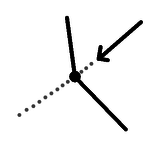In other words, a planar, connected, Eulerian graph (that is, has an Euler Cycle) can be drawn such that the situation here never happens. Here we are part way through drawing our graph, and we are approaching a vertex, only to find that we have to cross over a line that already exists.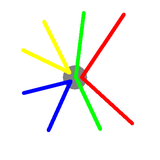So here's an example of what a vertex has to look like. We've exploded the vertex to show how the drawing proceeds. It doesn't matter which colours were drawn first, and it doesn't matter which direction we were travelling when we drew each colour, what matters is that they never cross each other during the drawing process.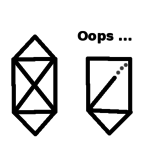Here is an example of a graph that can be drawn, and we are part way through an attempt to draw it which will fail. The theorem here claims that this never need happen.

So how do we prove this? Remarkably, the proof is almost identical to the usual proof that every graph such that every vertex has even degree is Eulerian, we just need to carry the extra condition with us.

### Proof

We proceed by induction.

Suppose $G$ is connected, planar, and every vertex has even degree. Suppose further that every graph satisfying those conditions but which has fewer edges can be drawn without crossing a previously drawn line.

Let $F$ be any face in $G$, and remove its edges, giving $G-F$. Certainly what is left is planar, and at every vertex it either has its original complement of edges, and so an even number, or two edges have been removed, so it's still an even number. So each component of $G-F$ is connected, planar, and has no odd degree vertices. So by our inductive hypothesis every component of $G-F$ can be drawn as required with no crossing lines.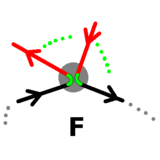Now begin drawing $F,$ proceeding clockwise. Whenever we visit a vertex $v$ in a component of $G-F$ not yet drawn, run around that (using our inductive hypothesis that we can draw it with no crossing lines) starting with the edge that is immediately clockwise from the edge in $F$ just drawn.

 This require some justification, but a few pictures of the different cases will soon convince the reader. A complete and detailed description of all the cases is more work than is really warranted.
When we return to $v$ we can continue around $F$ as required. By continuing around $F$ and ignoring vertices that have already been drawn we complete the entire traversal of $G$, as required.

It remains only to consider the induction base case(s). Ignoring the case of the single isolated vertex, the base case is a single vertex with a single loop, which clearly can be drawn without crossing any lines.

And so we are done.

## Using the new result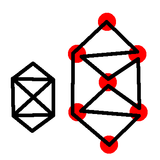Now we can draw our graph, but gently explode each vertex so that one pair of edges that come in then out as we draw them don't touch the other edges. Here's an example.

We can see now that our Euler Cycle is basically a distorted circle - it's a simple closed curve. As such, by the Jordan Curve Theorem (or in this case simply by common sense) we can see that it has an inside and an outside.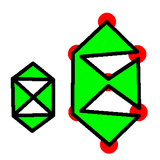Colour the inside one colour, and the outside the other colour. This gives us a bi-colouring of the original planar graph.

Thus we have shown that any connected, planar graph with an Euler Cycle can be two-coloured. That is, its dual is bi-partite.

And we're done.

 <<<< Prev <<<< When Obvious Is Not Obvious : >>>> Next >>>> Factoring Via Graph Three Colouring ...

 You should follow me on twitterI've decided no longer to include comments directly via the Disqus (or any other) system. Instead, I'd be more than delighted to get emails from people who wish to make comments or engage in discussion. Comments will then be integrated into the page as and when they are appropriate.

If the number of emails/comments gets too large to handle then I might return to a semi-automated system. That's looking increasingly unlikely.

# ContentsSuggest a change ( <-- What does this mean?) / Send me email